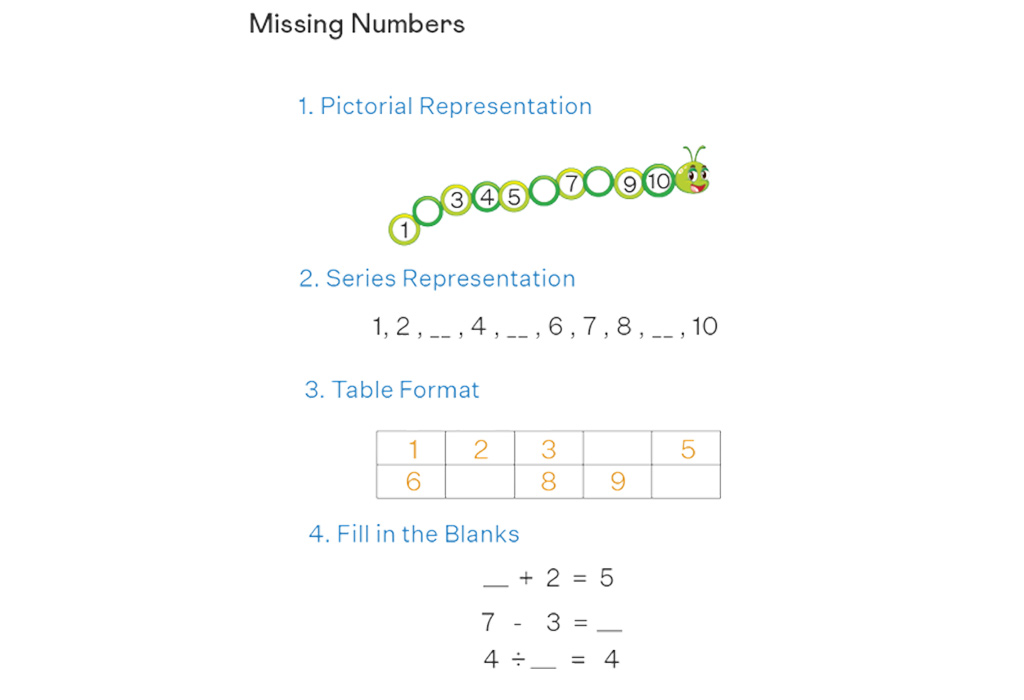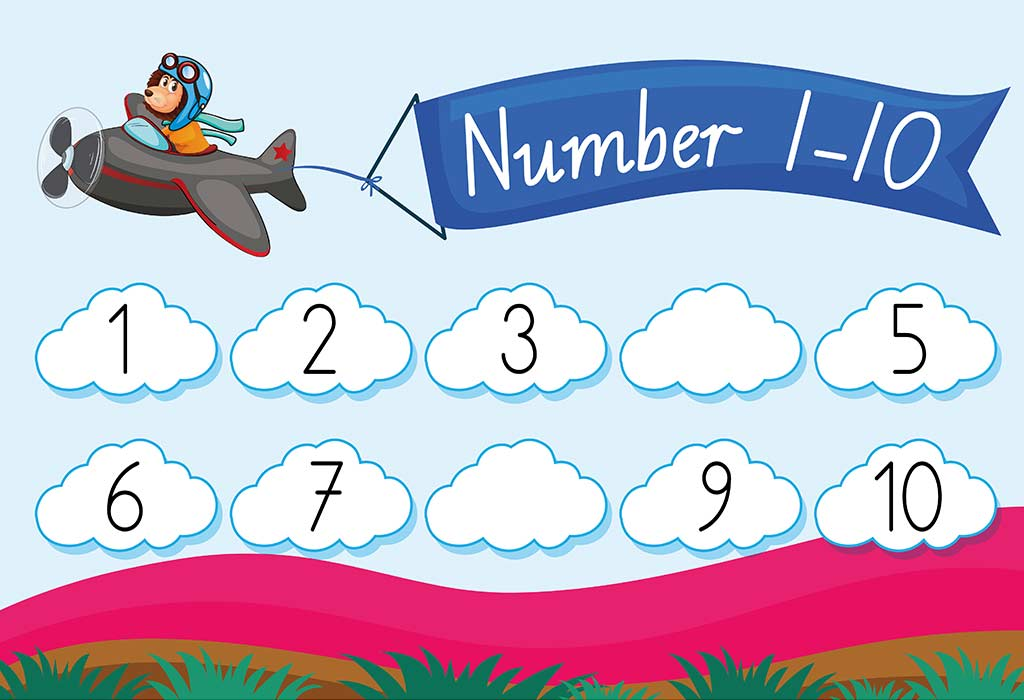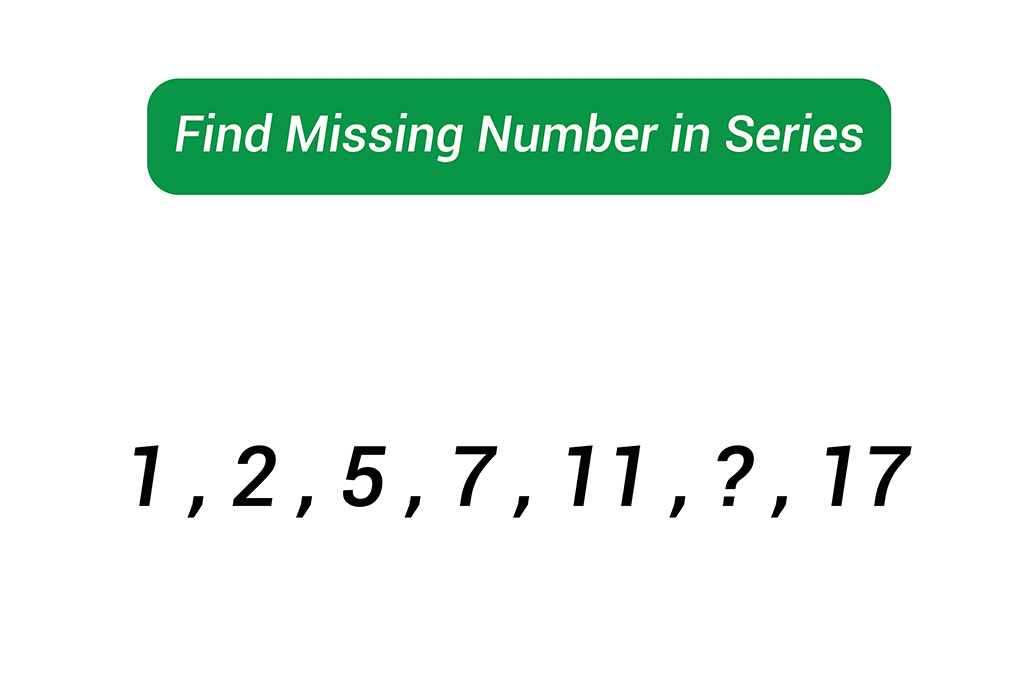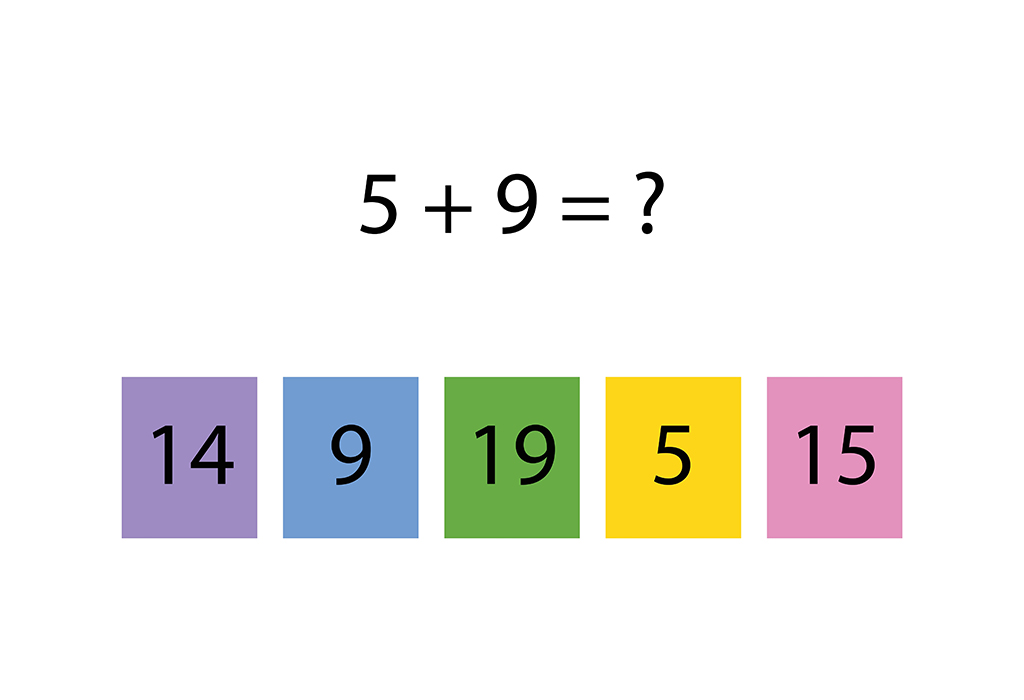HomeBig KidLearning & EducationMissing Numbers For Preschooler And Kids To Improve Math Skills

# Missing Numbers For Preschooler And Kids To Improve Math Skills

Maths is all about sharpening the problem-solving skills of children. Missing numbers or finding the missing values are a big part of helping children build problem-solving skills. Further, finding the missing numbers is a fun activity for the children to identify the patterns and even learn how to count.

In this article, we have provided information on what are missing numbers, advantages of learning to find missing numbers for children, different activities to learn how to find the missing numbers, and some solved examples on missing numbers for children. Let’s read!

## What Are Missing Numbers?

In simple words, missing numbers are the numbers that may have been missed in a given sequence or series. These missing numbers can be found by identifying the pattern of the numbers. When we are given a series with some blanks in between, there are a few numbers that have been missed. Children need to fill in these blanks with a suitable number after carefully analysing the pattern. The missing number method is great for memorising counting and multiplication tables and learning the use of various athematic operations.

## How To Find Missing Number In Series?

Wondering how to teach your little one to find the missing number in a sequence or series? We have an answer for you.

To find the missing digits in a particular sequence or series, we have to first identify the pattern of the sequence or series. This pattern can be anything, and we need to apply all possible mathematical operations to find the pattern in the given question. Let us take a look at the series of counting numbers from 1 – 10, 1 – 20, and 1 – 50.

### Missing Numbers From 1-10

Here we have written the series of counting numbers from 1 – 10 with a few blanks. Children need to fill in the missing numbers by identifying the pattern.

1, 2, _, 4, _, 6, 7, _, 9, 10.

In the above series, there are some blanks, and a certain pattern is being followed here. This pattern is of counting numbers from 1 – 10. Let your child count and then fill in the missing numbers in the series.

The answer will be: 1, 2, 3, 4, 5, 6, 7, 8, 9, 10.

### Missing Numbers From 1-20

Here we have written the series of counting numbers from 1 – 20 with a few blanks. Children need to fill in the missing numbers by identifying the pattern.

1, 2, _, 4, _, 6, 7, _, 9, 10, 11, _, _, 13, _, 15, 16, _, 18, _, 20.

In the above series, there are some blanks, and a certain pattern is being followed here. This pattern is of counting numbers from 1 to 20. Let your child count and then fill in the missing numbers in the series.

The answer will be: 1, 2, 3, 4, 5, 6, 7, 8, 9, 10, 11, 12, 13, 14, 15, 16, 17, 18, 19, 20.

### Missing Numbers From 1-50

Here we have written the series of counting numbers from 1 – 50 with a few blanks. Children need to fill in the missing numbers by identifying the pattern.

1, 2, _, 4, _, 6, 7, _, 9, 10, 11, _, _, 13, _, 15, 16, _, 18, _, 20, 21, _, 23, 24, 25, _ 27, 28, _, 30, 31, 32, 33, _, 35, _, 37, 38, 39, _, _, 42, 43, _, 45, _, 47, _, _, 50.

In the above series, there are some blanks, and a certain pattern is being followed here. This pattern is of counting numbers from 1 to 50. Let your child count and then fill in the missing numbers in the series.

The answer will be: 1, 2, 3, 4, 5, 6, 7, 8, 9, 10, 11, 12, 13, 14, 15, 16, 17, 18, 19, 20, 21, 22, 23, 24, 25, 26, 27, 28, 29, 30, 31, 32, 33, 34, 35, 36, 37, 38, 39, 40, 41, 42, 43, 44, 45, 46, 47, 48, 49, 50.

## Missing Number In Addition And Subtraction

Now, we will take a look at how to solve the questions of the missing number in addition and subtraction with the help of examples. Let’s begin.

1. _ + 5 = 9

Solution: We have to find a number that, when added to 5, gives the sum equal to 9.
When we add 4 to 5, the sum is equal to 9.
Therefore, 4 + 5 = 9.

2.  3 – 1 = _

Solution: We have to find a number that is equal to 3 minus 1.
We can solve this using a simple subtraction operation.
3 -1 = 2
Therefore, 3 – 1 = 2.

3. 10 – _ = 6

Solution: We have to find a number which, when subtracted from 10, gives the difference equal to 6.
10 – 4 = 6
Therefore, 10 – 4 = 6.

4. 7 + 4 = _

Solution: We have to find a number that is equal to 7 plus 4.
7 + 4 = 11
Therefore, 7 + 4 = 11

## Missing Number In Before And After

In this section, we will look at how to solve missing numbers in before and after with the help of examples. Let’s begin!

1. _, 7, _

Solution:The number before 7 is 6, and the number after 7 is 8.
So, the sequence will be: 6, 7, 8.

2. _, 20, _

Solution: The number before 20 is 19, and the number after 20 is 21.
So, the sequence will be: 19, 20, 21.

3. _, 43, _

Solution: The number before 43 is 42, and the number after 43 is 44.
So, the sequence will be: 42, 43, 44.

4. _, 77, _

Solution: The number before 77 is 76, and the number after 77 is 78.
So, the sequence will be: 76, 77, 78.

## Missing Number In Multiplication And Division For Kids

In this section, we will look at how to solve missing numbers in multiplication and division with the help of examples. Let’s begin!

1.  3 × 2 = _

Solution: The product of 3 and 2 is equal to 6.
So, 3 × 2 = 6

2. 5 x _ = 10

Solution: We have to find a number that, when multiplied by 5, gives the answer equal to 10.
Therefore, 5 x 2 = 10

3. 10/2 = _

Solution: When 10 is divided by 2, the quotient is 5.
Therefore, 10/2 = 5

4. _/6 = 2

Solution: When 12 is divided by 6, the quotient is equal to 2.
So, 12/6 = 2.

## Missing Number Activity For Children

Below we have covered a math missing number activity for children that will help kids to understand how to fill in the missing numbers. This is a fun activity that will keep your little one entertained while learning an important mathematical concept.

1. Take popsicle sticks and write the number sequence on them. For example, you can write: 2, 4, 6, 8, 10, 12, 14, 16, 18, 20.
2. Make sure to leave a few blanks for the kids to fill in the missing numbers. For example, 2, _, 6, 8, _, 12, 14, _, 18, 20.
3. Take a few clothespins and write the missing numbers on them. For example, 4, 10, 16.
4. Hand over these clothespins to your child and ask them to put them in the right place on popsicle sticks to complete the number sequence.

####### 1. Fill In The Missing Numbers

Kids love to write on walls, floors, etc. Use this habit as a teaching opportunity, and using chalk, write a few number series on the floor, leaving a blank for a few numbers. Ask your child to fill in the missing numbers by observing the pattern.

You can use the following number series:

####### 2. Magnetic Numbers

Get a pack of magnetic numbers. On a board, write a number sequence, leaving blank spaces for a few numbers. You can use the following number sequence:

###Ask your child to fill in the blank numbers using magnetic numbers.

###Give your child simple addition, subtraction, multiplication and division missing number problems to solve.

#### 4. Learn With Building Block

Take a few building blocks and mark numbers on them as per the number sequence. For example, you can follow this number sequence:

2, _, 6, 8, _, 12.

Line up the building blocks and ask your child to write the missing numbers on the building blocks as per the pattern.

The missing numbers that they need to fill will be: 4, 10.

### Advantage Of Missing Number Activities

Wondering why your child must learn missing numbers with the help of activities? The activity of finding missing counting numbers can help your little one to develop mental skills and enhance their fine motor practice of learning. It also helps kids to understand important mathematical operations like addition, subtraction, multiplication, and division.

## Solved Problems On Missing Number For Kids

Here are some missing number questions with solutions for children to understand the concept of missing numbers easily.

1. Find the missing number in the series: 30, 20, _, 0.

Solution: We can see the numbers in the above sequence are in decreasing order. The difference between the numbers 30 – 20 = 10.

Therefore, the numbers have a difference of 10 and are decreasing in order. Therefore, by subtracting 10 from 20, we get 10, which is the missing number in the series.

Therefore, the missing number is 10.

2. Find the missing number: _/3= 3

Solution: Here we have to find a number when divided by 3, which gives the answer 3.

So, 9/3 = 3.

3. Solve to find the missing number: 3 + 7 = _

Solution: Here we have to find a number which is equal to 3 plus 7.

So, 3 + 7 = 10

4. Find the missing numbers in the series: _, 6, _, 12, 15 .

Solution: We can see the numbers in the above sequence are in ascending order. The difference between the numbers 15 – 12 = 3.

Therefore, the numbers have a difference of 3 and are in ascending order. Therefore, by subtracting 3 from 6, and 12 we get 3 and 9 respectively, which are the missing number in the series.

Therefore, the missing number are 3 and 9.

## Practice Questions On Missing Numbers

1. What is the missing number in the following sequence?
3, _, 7, 9, 11

1. 4
2. 5
3. 6

2. Find the missing number in the pattern given below: 5, 10, _, 20, 25

1. 15
2. 30
3. 17

Missing numbers is an essential mathematical concept that kids must be taught at an early age. Learning this concept helps children to understand important maths operations and also learn how to identify patterns. We hope this article helped your kid to learn how to solve and fill in missing numbers. Make sure to make your child practice this with the help of activities mentioned in the article. If you child does not show interest in learning, give them some time and teach again. But don’t force them to learn. Let them take their own time!

RELATED ARTICLES